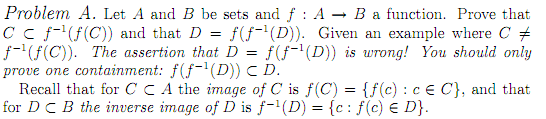# Linear Maps

## Homework Statement## The Attempt at a Solution

I don't know how to start a proof for this. Intuitively I would think think that C = f^(-1)(f(c)), which would imply that C is a subset of f^(-1)(f(c)), however that is not the case and the problem asks for an example when that is not true. Does this mean that f(C) sends all elements c of C from A to B and that f^(-1) sends all elements c of C from B to A?

lanedance
Homework Helper
its good to start with small discrete sets and see if you can find a good example

how about considering A = {a,b} both mapped to the same point f(a) = f(b) = d

Thanks I kind of figured it out. One questions would a differentiable map be considered an example where if you have f(a) = d then then f^(-1)(f(a)) wouldn't necessarily equal a?

lanedance
Homework Helper
I'm not sure why you would need to consider differntiability? You;re just looking at maps between sets

the example I gave in post #2 should be sufficient...